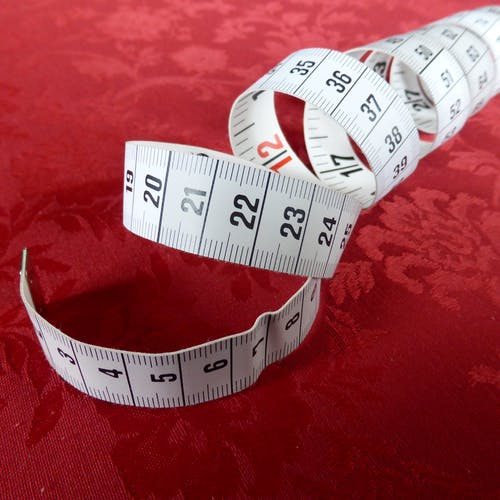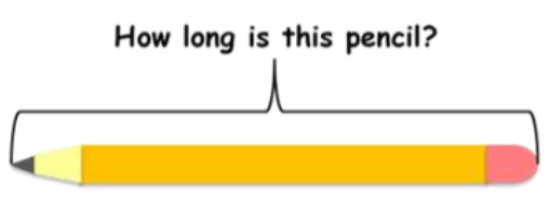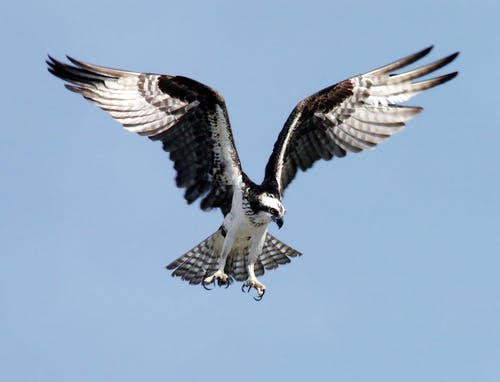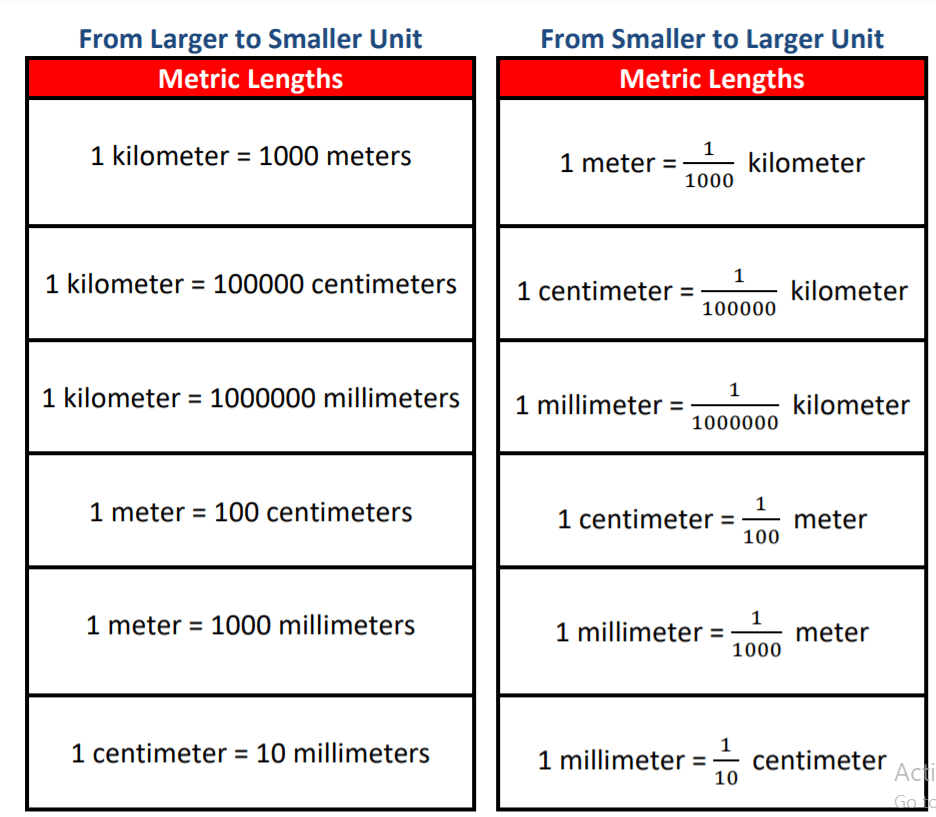# The Metric Units of Length

 Listen to this Lesson:

When fourth graders learn about conversion and measurements, they usually start with the metric units of length. They become fluent in determining the different metric units of length and how they are related to each other, as well as in converting larger units to smaller units and vice versa.

Math teachers can transform these early lessons on conversion and measurements into a fun learning experience by incorporating diverse teaching strategies in the classroom. To help out, we compiled a list of such strategies. Read on and learn more.## Strategies to Teach the Metric Units of Length

### Length

You can start your lesson by explaining what length is. You can define it as a measurement that determines how long an object is from one of its ends to the other. This can also mean the distance between one object and/or place to another.

You can provide relatable examples. For instance, ask students to look at their pencils and think of their length. You can also ask them to observe a building outside and try to think how long it is from one of its ends to the other.### Units of Length

Explain to students that there are different units to measure different types of length. Point out that the basic unit of length is called meter. Present the other units of length by writing them on the whiteboard and arranging them from the smallest to the largest. That is:

• millimeter
• centimeter
• decimeter
• meter
• decameter
• hectometer
• kilometer

#### Picking the Best Unit of Measure

Students will also learn how to select the best unit of measure for any given object. Highlight that the most frequently used units of measure are: millimeter, centimeter, meter, and kilometer. Explain which type of unit is used in which situations and for which objects.

Point out that we use millimeters when we’re measuring tiny objects that are less than one centimeter. You can also prepare images of super tiny insects and specimens to provide examples. We use the symbol mm for millimeters.Then, underline that we also use centimeters for measuring small, but not super tiny objects. For example, if something is less than the size of a ruler, then using centimeters is best. We use the symbol cm for centimeters.

Proceed with explaining in which situations we use meters. Highlight that we use them to measure medium-sized objects that are more than the length of a ruler. For example, if we want to know how far our living room is from our bedroom, we’ll use meters. The symbol is m.

Finally, explain that we use kilometers for long distances. For instance, if we want to know how far one city is from another or if we want to measure the distance of the Earth from the sun, we’ll use kilometers. The symbol is km.

##### Reflection:

After you’ve explained which units of length are used for which objects/in which situations, you can write down several examples of random objects/situations on the whiteboard and ask students to reflect on them and determine whether we will use meters, kilometers, centimeters or millimeters to measure them. For example:

• shoe size
• the distance between Earth and Saturn
• dining table
• a seed
• the thickness of a dime
• the distance from New York to Los Angeles
• the wingspan of an eagle### The Relationship Between the Metric Units of Length

Point out that the different units of length are related to one another. For units larger than one meter, we use the following prefixes: kilo-, hecto-, and deca-. By using them, we can describe distances and lengths that are larger than a meter. More specifically:

• 1 kilometer = 1, 000 meters
• 1 hectometer = 100 meters
• 1 decameter = 10 meters

When it comes to units smaller than a meter, we use the prefixes: deci-, centi-, and milli-, through which we can describe distances and lengths that are smaller than a meter. In more specific terms:

• 1 decimeter = 1/10 meters
• 1 centimeter 1/100 meters
• 1 millimeter = 1/1,000 meters

### The Metric Length Conversion Chart

The different metric lengths can be converted from one unit to another. You can create a conversion chart and place it on a wall in the classroom or simply write one on the whiteboard:### Conversion of Measurement of Lengths

#### Converting Larger Units to Smaller Units

Explain to students that to convert a larger unit to a smaller unit, we need to multiply. How do we know by how much we should multiply? First, we’ll check the above chart and see how many smaller units are required to make one larger unit.

Once we know this number (and we know it by checking the above chart and later on we remember it by heart), we’ll simply multiply this number by the number of larger units. Provide an example, such as:

5 km equals how many meters?

Since we know that 1 km equals 1,000 m, we will use this as our starting point:

1 km = 1,000 m

5 km = 5 1,000 = 5,000 m

5 km = 5,000 m

Make sure to provide an example of converting larger units to smaller units that involves more than one step. For instance, let’s say we want to change kilometers to centimeters. Based on what we know so far, first, we’ll change the value of kilometer to meters and then to centimeters.

However, a faster way to do this is to perform the conversion in one step. We can jump from kilometers and land to centimeters right away. Since we know that 1 km = 1,000 m, and 1 m = 100 cm, we can directly multiply by 100, 000 (because 1,000 100 = 100, 000.

For instance, 4 km equals how many centimeters?

1 km = 100, 000 cm

4 km = 4 × 100, 000 = 400, 000 cm

4 km = 400, 000 cm

#### Converting Smaller Units to Larger Units

Explain to students that when it comes to converting smaller units to larger units, we have to divide them by the number of smaller units that are required to make the larger unit. Again, we’ll check the above chart to see how many smaller units are required to make one larger unit.

Provide an example of converting smaller units to larger units, such as:

100 millimeter equals how many centimeters?

To find the answer to this, we’ll check the chart and see how many smaller units are needed to make a larger unit. In this case, we want to see how many centimeters 1 millimeter equals to. We can observe that 1 mm is one-tenth of 1 cm. So, we’ll divide the value of millimeters by 10.

100 mm = ___cm?

1 mm = 1/10 cm

100 mm = 100/10 = 10 cm

100 mm = 10 cm

Provide an example of converting smaller units to larger ones that involves more than one step. Let’s say we want to convert mm to km. This involves two conversions – first, we’ll change the value of millimeter to centimeter, and only then to meter.

We can also change millimeters to meters in just one step. We can jump from millimeters and land to meters right away. To convert from mm to cm we need to divide by 10, and from cm to m by 100. With this in mind, we can directly divide by 1,000 (as 10100 = 1,000).

For example:

1,000 mm = __m?

We’ll divide the value of millimeters by 1,000:

1 mm = 1/1,000 m

1,000 mm = 1,000/1,000 m = 1 m

1,000 mm = 1 m

If you have the technical possibility in your classroom, feel free to enrich your lesson on the metric units of length with multimedia materials, such as videos. For instance, use this video to help students master conversion from larger units to smaller ones and vice versa.

In addition, this video by Khan Academy contains worked-out examples of how to convert larger units to smaller units, such as kilometers and centimeters to millimeters. Play these videos in your classroom and let children discuss them.

## Activities to Practice the Metric Units of Length

### Convert to Smaller Units Activity

This is an online activity where children will get to practice their ability to convert larger to smaller units. To use this activity in your classroom, make sure you have enough technical devices (one per student).

Explain to students that they will be presented with several math problems, in each of which they will have to convert one metric unit to another one. This is an individual activity, which makes it a good match for parents who are homeschooling their children as well.

### Conversion Matching Game

In this game, students will practice their knowledge of how the different metric units of length are related to each other, and more specifically how to convert larger units to smaller units, as well as smaller to larger ones.

To implement this game in your classroom, you’ll need to create two sets of cards, one pink and one blue. The pink set contains cards that should be matched with the cards from the blue set, based on the values written on them.

For instance, if there is a pink card with 0.25 kilometers written on it, make sure there is a blue card with a corresponding value, but expressed in a different metric unit, such as 250 meters. Make sure there is exactly one match per card, to avoid confusion.

Divide students into groups of 3 or 4 and provide instructions for the game. Explain that the members of a group work together in order to match the pink cards with the blue cards as quickly as they can. The group that has all cards matched correctly first wins the game.

## Before You Leave…

This article outlined a few useful strategies on how to teach the metric units of length to fourth graders. If you liked these tips and you’re interested in more math resources for children of all ages, sign up for loads of free content!

You can also check out our blog, where you’ll find plenty of awesome materials! And if you’re ready to become a member, simply join us in the MathTeacherCoach!